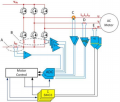# PMSM Torque Determination from Winding Current

#### transconductance

Joined Jun 29, 2019
80
I am trying to measure the torque being delivered by a permanent-magnet synchronous motor aka AC servo motor by measuring the current. This is a motor with a three-phase stator and a permanent-magnet rotor that's wound to be excited with a three-phase sinusoid.

I can find the answer to this all over the web but no-place that gives me enough specifics to actually make the measurement.

I know that torque is (more or less) proportional to current. The motor data sheet gives me a torque constant of 0.62 Nm per amp. So far, so good, but which amps? Is that the instantaneous peak DC current in one winding with the others unexcited? Is it the sum of the instantaneous DC currents? Is it the instantaneous current in the q axis as projected from the shaft angle? Is it the RMS current? If so, is it the RMS current in one wire or in one winding (which is not necessarily the same in a delta-wound stator)?

Short version: I clamp an oscilloscope current probe on one wire and read the current waveform. How do I get from here to torque? The current is approximately sinusoidal with a peak of about 6.3 amps. The RMS current, as computed by the 'scope is about 4.8 amps.

#### nsaspook

Joined Aug 27, 2009
11,312
The instantaneous current in the q axis as projected from the shaft angle (Clarke transform) is the current value to use. In order to get that current you need to measure at least two (you can calculate the third) phases of the motor currents. Those measurement can be implemented at several places in the power chain with inverter leg shunt resistors being a common implementation.

https://www.analog.com/en/technical...techniques-for-industrial-motion-control.htmlFigure 4 illustrates the various current feedback options described above. While only one of these options is required for control feedback, the dc link current signal may be used as a backup signal for protection.

As DC motor, indirectly determine and control Φ and I to control motor torque. The flux and the current have direct proportion with motor torque. Changes the current will change the motor torque. It’s very simply. But as AC motor, the motor torque control not only need I and Φ, the φ is also important. The φ will change with the rotor current frequency. The Φ come from stator current and rotor current, it will change along with the motor load change, so AC motor in dynamic running, it’s controlled more difficult than DC motor. AC motor should have the parameter relation like the DC motor. Transvector Control could simulate AC motor into DC motor, and simply the control. The base theory: Use the 3-phase AC motor rotating magnetic field transform into like DC motor 2-phase rotating magnetic field, then control the 2-phase current to control the torque.

#### transconductance

Joined Jun 29, 2019
80
OK, so I am looking at one phase waveform, so maybe we can simplify this. Suppose I pick the peak of this current waveform.
Can't I then assume that the other two are equal? And so should each be half of the measured peak and have the opposite
sign? Also at this point, the angle input to the Clarke transform should be zero degrees.

Now here's something I'm not sure about. Do I need to divide the phase leg currents by sqrt(3) to get the
winding currents or does Clarke take care of that? I think Clarke takes care of that. Certainly that matrix
has a couple if sqrt(3) terms in it...

Anyway, selecting the measurement at 0 degrees greatly simplifies things and the result after some hand-waving (and a spreadsheet) I get that, at zero degrees, the quadrature current is numerically the same as the measured current.

I measured 6 amps, so I'm guessing the other two phase currents are each -3 amps. My q current is therefore
also 6 amps and my torque is 3.7 Nm

#### mcardoso

Joined May 19, 2020
226
So I am admittedly short on the mathematics to directly answer you question above, however I did support AC PM servomotors and drives for a number of years while working for an industrial automation supplier. Motor amps is the best measurement you will get of motor torque without an external torque transducer, however be aware that due to manufacturing tolerances, the torque constant provided on the motor data sheet is representative of the product family, but will not perfectly characterize the individual motor you are using. It should be close (<3%) however this might not be acceptable for high accuracy applications like torque wrenches. Additionally the torque constant will vary in the motor depending on many factors like motor amps drawn, rpm, temperature, and possibly age of the motor as well. For 99% of applications, these additional factors do not matter and motor amps can be directly equated to motor shaft torque.